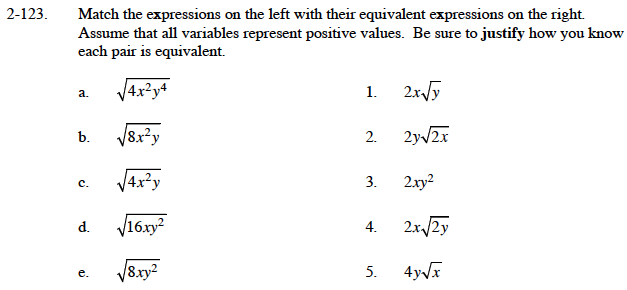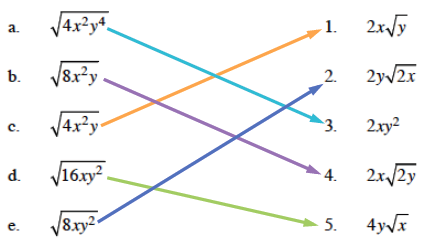### Home > AC > Chapter 13 > Lesson 13.RE1-S > Problem2-123

2-123.Simplify the expressions in the right column. For example:

$\sqrt{8\textit{xy}^2} = \sqrt{4 \cdot 2 \cdot \textit{x} \cdot \textit{y}^2}=\sqrt{4} \cdot \sqrt{\textit{y}^2} \cdot \sqrt{2\textit{x}}$

Take the square roots of 4 and y2.